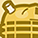# QlikView App Dev

Discussion Board for collaboration related to QlikView App Development.

Announcements
QlikWorld 2023, a live, in-person thrill ride. Save \$300 before February 6: REGISTER NOW!
cancel
Showing results for
Did you mean:Contributor II

## How to do the following: sum(sum(Field A) * avg(Field B)) in Qlik?

Hi,

I have two fields: A and B. I need to sum all the values in Field A and multiply it by the average value of Field B so basically sum(sum(Field A) * avg(Field B)). When im trying to do this in qlik I get an error. How could I achieve this? Thanks in advance.

Labels (2)

• ### Scripting

1 Solution

Accepted SolutionsMVP & Luminary

It's difficult to say what might be the cause. Thinkable would be that [Field A] and [Field B] have no proper association to each other respectively in regard to the Year and Month.

To get more insights you may use a table-chart with Month as dimension and the following expressions:

sum({\$<Year={"\$(=max(Year)-1)"}>} [Field A])
sum([Field A])
avg({\$<Year={"\$(=max(Year)-1)"}>} [Field B])
avg([Field B])
sum({\$<Year={"\$(=max(Year)-1)"}>} [Field A]) * avg({\$<Year={"\$(=max(Year)-1)"}>} [Field B])

Again everything showed the expected results?

- Marcus

10 RepliesMVP & Luminary

Nesting aggregations could be done with aggr() to specify the needed dimensionally context for the calculation. This means something like:

sum(aggr(sum(Field A) * avg(Field B), Dim1, Dim2))

- MarcusContributor II
Author

Thanks, can I use Field A as Dim1 and Field B as Dim2?MVP & Luminary

It depends - but rather not. Field A/B are usually measures likes Sales or Amount and Dim 1/2 are normally dimensions like Products or Stores or Periods - mostly the dimensions from the chart and/or a more granular ones - for example if the calculation should be done on a date-level but the chart displayed only YearMonth - and sometimes also on any of the made selections.

- MarcusContributor II
Author

I see, im trying to get this to work with previous year so that the expression would be calcualted based on previous year's values and it would be used in a dashboard / text object (not a table). So far I have this:

sum({\$<Year={\$(=Year-1)}, Month=>}aggr(sum(Field A) * avg(Field B), Year, Month))

but it shows 0?MVP & Luminary

I think I would try it with:

sum(aggr(
sum({\$<Year={\$(=max(Year)-1)}, Month=>} [Field A]) *
avg({\$<Year={\$(=max(Year)-1)}, Month=>} [Field B]),
Year, Month))

- MarcusContributor II
Author

Thank you for the suggestion. Unfortunately it's still showing 0.MVP & Luminary

In such a case I would check each single part, like:

sum({\$<Year={"\$(=max(Year)-1)"}, Month=>} [Field A])
\$(=max(Year)-1)
avg({\$<Year={"\$(=max(Year)-1)"}, Month=>} [Field B])

Does all work like expected?

- MarcusContributor II
Author

Hi, I checked all parts and they show correct values. Problem comes while multiplying them for some reason.MVP & Luminary

It's difficult to say what might be the cause. Thinkable would be that [Field A] and [Field B] have no proper association to each other respectively in regard to the Year and Month.

To get more insights you may use a table-chart with Month as dimension and the following expressions:

sum({\$<Year={"\$(=max(Year)-1)"}>} [Field A])
sum([Field A])
avg({\$<Year={"\$(=max(Year)-1)"}>} [Field B])
avg([Field B])
sum({\$<Year={"\$(=max(Year)-1)"}>} [Field A]) * avg({\$<Year={"\$(=max(Year)-1)"}>} [Field B])

Again everything showed the expected results?

- MarcusCommunity Browser